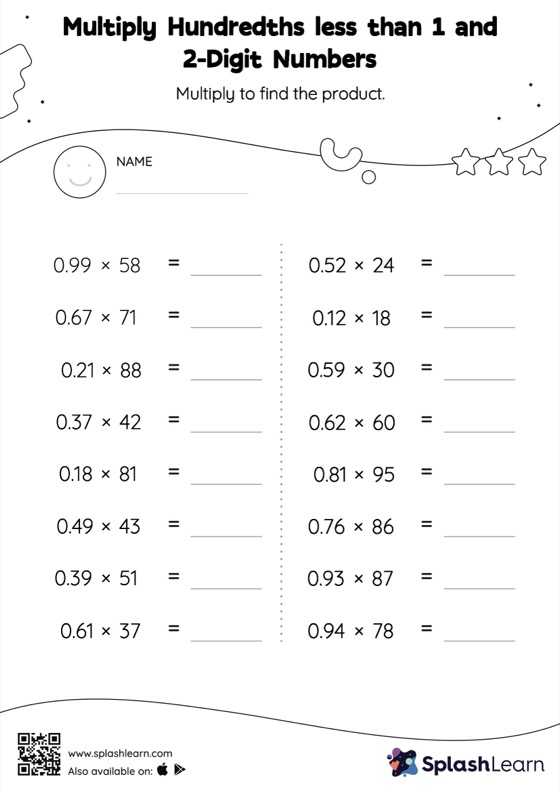# Multiply Hundredths less than 1 and 2-Digit Numbers: Horizontal Multiplication Worksheet

Home > Multiply Hundredths less than 1 and 2-Digit Numbers: Horizontal MultiplicationWhen multiplying a decimal by a two-digit number, students may ignore the decimal point during calculations and bring it back when writing the result. This multiply hundredths less than 1 and 2-digit numbers worksheet provides plenty of practice with this concept. As the worksheet uses a horizontal format, it allows for more creativity when it comes to how the student solves the problem. In contrast, in the vertical format, students usually employ the standard method to solve.